Next: Closure of Attribute Sets Up: Functional Dependencies Previous: Basic Concepts

Closure of a Set of Functional Dependencies

1. We need to consider all functional dependencies that hold. Given a set F of functional dependencies, we can prove that certain other ones also hold. We say these ones are logically implied by F.
2. Suppose we are given a relation scheme R=(A,B,C,G,H,I), and the set of functional dependencies:

AB

AC

CGH

CGI

BH

Then the functional dependency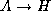is logically implied.

3. To see why, let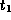and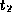be tuples such that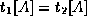As we are given AB , it follows that we must also have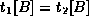Further, since we also have BH , we must also have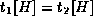Thus, whenever two tuples have the same value on A, they must also have the same value on H, and we can say that AH .

4. The closure of a set F of functional dependencies is the set of all functional dependencies logically implied by F.
5. We denote the closure of F by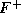.
6. To compute, we can use some rules of inference called Armstrong's Axioms:
• Reflexivity rule: ifis a set of attributes and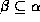, then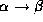holds.
• Augmentation rule: ifholds, and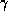is a set of attributes, then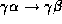holds.
• Transitivity rule: ifholds, and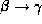holds, then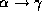holds.
7. These rules are sound because they do not generate any incorrect functional dependencies. They are also complete as they generate all of.
8. To make life easier we can use some additional rules, derivable from Armstrong's Axioms:
• Union rule: ifand, then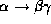holds.
• Decomposition rule: ifholds, thenandboth hold.
• Pseudotransitivity rule: ifholds, and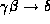holds, then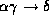holds.
9. Applying these rules to the scheme and set F mentioned above, we can derive the following:
• AH, as we saw by the transitivity rule.
• CGHI by the union rule.
• AGI by several steps:
• Note that AC holds.
• Then AGCG , by the augmentation rule.
• Now by transitivity, AGI .
(You might notice that this is actually pseudotransivity if done in one step.)Next: Closure of Attribute Sets Up: Functional Dependencies Previous: Basic Concepts

Osmar Zaiane
Tue Jun 9 15:12:55 PDT 1998